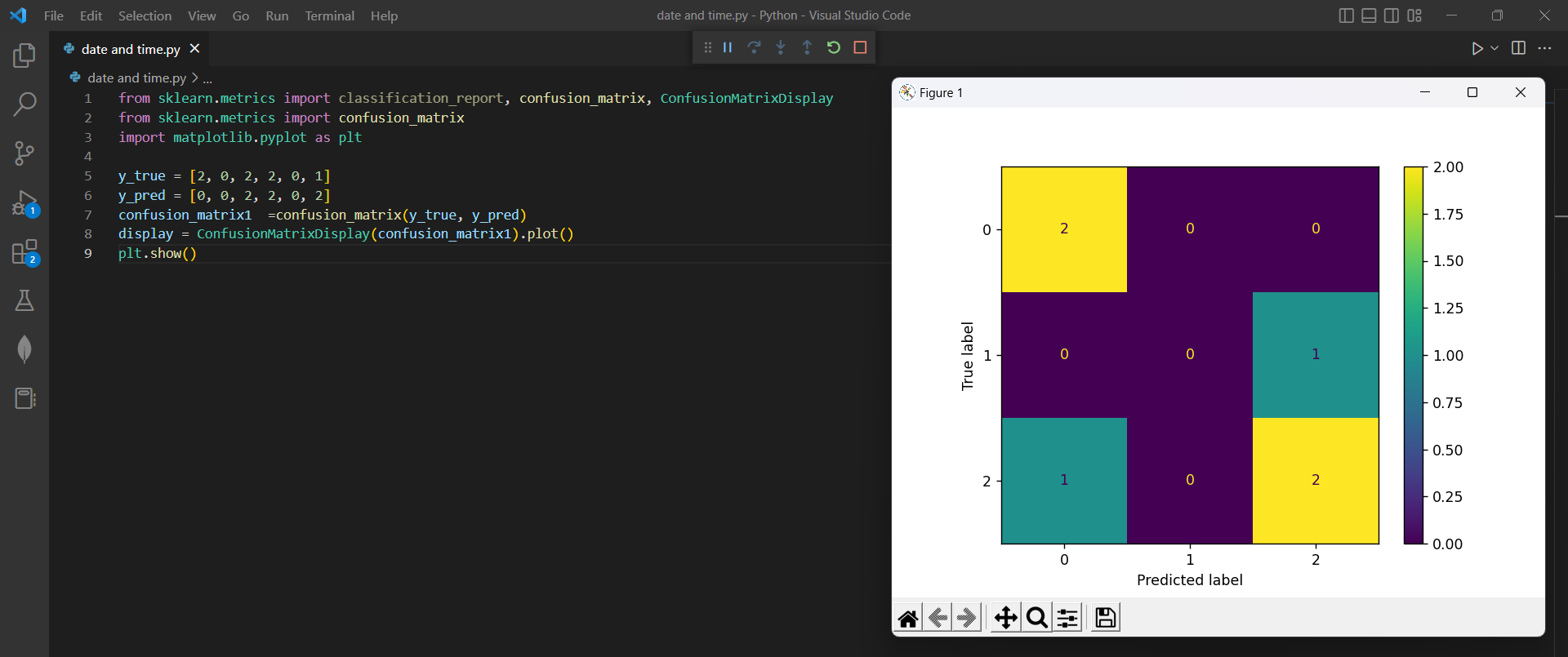# How to Visualize Confusion Matrix using Scikit-Learnby vigneshchennai74Updated: Feb 15, 2023Solution Kit

This program is calculating and visualizes a confusion matrix for a classification problem using the Scikit-Learn library in Python. The confusion matrix is a table that summarizes the performance of a classification algorithm by showing the number of true positives, true negatives, false positives, and false negatives for each class.

The confusion_matrix() function from scikit-learn takes the y_true and y_pred arrays as inputs and calculates a confusion matrix, which shows the number of true positives, true negatives, false positives, and false negatives for each class.

The ConfusionMatrixDisplay() function from scikit-learn takes the confusion matrix as input and creates a plot of the matrix. The resulting plot displays the confusion matrix as a grid of colored squares, where the color of each square represents the number of data points that fall into that category.

The plt.show() function displays the plot on the screen. Matplotlib is used to plot the confusion matrix generated by the Scikit-Learn library. The ConfusionMatrixDisplay() function from Scikit-Learn creates a plot of the confusion matrix using Matplotlib.

This helps users to evaluate the performance of a classification algorithm and understand its strengths and weaknesses.Preview of the output that you will get on running this code from your IDE

### Code

In this solution we have used Sklearn library.

1. Copy the code using the "Copy" button above, and paste it in a Python file in your IDE.
2. Run the file to get the output

I hope you found this useful. I have added the link to dependent libraries, version information in the following sections.

I found this code snippet by searching for "ConfusionMatrixDisplay(confusion_matrix).plot() doesn" in kandi. You can try any such use case!

### Environment Tested

I tested this solution in the following versions. Be mindful of changes when working with other versions.

1. The solution is created in Python 3.7.15 version
2. The solution is tested on scikit-learn 1.0.2 version

Using this solution, we are able to plot the confusion matrix using Scikit learn library in Python with simple steps. This process also facilities an easy to use, hassle free method to create a hands-on working version of code which would help us plot a confusion matrix in Python.

### Dependent Library

scikit-learnby scikit-learn

Python54382Version:1.2.2scikit-learn: machine learning in Python

Support
Quality
Security
Reuse

scikit-learnby scikit-learn

Python54382Version:1.2.2License: Permissive (BSD-3-Clause)

scikit-learn: machine learning in Python
Support
Quality
Security